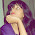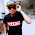## Tuesday, April 21, 2015

### Arduino Nano: Capture analog input in Timer Interrupt

Last post show a "oscilloscope-like waveform on 0.96" 128X64 I2C OLED with Arduino Nano". But the analog input is captured inside loop(), it's not in fixed timing, and affected by the slow operation of displaying.

To capture analog input in accurate timing, we are going to implement TIMER1 ISR (Interrupt Service Routine) in this example, and call analogRead() to capture analog input inside ISR.

Connecttion between Arduino Nano and OLED, refer to last post.

To understand Arduino Timer and Interrupt, it have a good tutorial HERE.

I2C_OLED_scope.ino
``````// Display analog input to mini-OLED I2C,
// capture input inside TIMER1 ISR
// http://arduino-er.blogspot.com/

// OLED display: ref u8glib: https://code.google.com/p/u8glib/
// To install u8glib on Arduino IDE: http://goo.gl/j3olBA
#include "U8glib.h"

U8GLIB_SSD1306_128X64 u8g(U8G_I2C_OPT_NONE|U8G_I2C_OPT_DEV_0);

const int WIDTH=128;
const int HEIGHT=64;
const int LENGTH=WIDTH;

const int LED = 13;
boolean LEDst = false;

//true: request capture analog input in ISR
//false: stop capture, draw waveform in loop
boolean capture = false;

const int analogInPin = A0;
int analogInValue = 0;

int x;
int y[LENGTH];

/*
reference: Arduino Timer and Interrupt Tutorial
http://blog.oscarliang.net/arduino-timer-and-interrupt-tutorial/

For our TIMER1 Interrupt:
Clock Freq = 16MHz
no prescale, 1
16MHz - 0.0625us/cycle

To calculator preload value to generate 1ms(1KHz)
(65536 - t) x 0.0625us = 1000us
t = 65536 - 1000/0.0625 = 49536

To calculator preload value to generate 0.5ms(2KHz)
(65536 - t) x 0.0625us = 500us
t = 65536 - 500/0.0625 = 57536

*/
const int TCNT1_PRELOAD = 57536;

void clearY(){
for(int i=0; i<LENGTH; i++){
y[i] = -1;
}
}

void drawY(){
u8g.drawPixel(0, y);
for(int i=1; i<LENGTH; i++){
u8g.drawLine(i-1, y[i-1], i, y[i]);
}
}

void setup(void) {

pinMode(LED, OUTPUT);

// initialize Timer1
noInterrupts(); // disable all interrupts
TCCR1A = 0;
TCCR1B = 0;
TCNT1 = TCNT1_PRELOAD;
TCCR1B |= (1 << CS10);   // no prescaler
TIMSK1 |= (1 << TOIE1); // enable timer overflow interrupt

x = 0;
clearY();
capture = true;

interrupts(); // enable all interrupts
}

void loop(void) {
if(!capture){

u8g.firstPage();
do {
drawY();
} while( u8g.nextPage() );

//start capture another frame
x = 0;
clearY();
capture = true;
}

delay(100);

}

ISR(TIMER1_OVF_vect){
TCNT1 = TCNT1_PRELOAD; // preload timer

if(capture){
//toggle LED
digitalWrite(LED, LEDst=!LEDst);

analogInValue = analogRead(analogInPin);
y[x] = map(analogInValue, 0, 1023, HEIGHT-1, 0);

x++;
if(x >= WIDTH){
capture = false;
}
}
}
``````

#### 2 comments:

1.i tried to display the ecg signal using this program but the output wasn't as expected. what i mean to say is that is there any way to shrink the display wave horizontally. And also i wanted to know that is it possible to display more than one waves on oled and some numbered values along with them?

1.i currently having the same problem for my project. Have you found the solution and helps me?